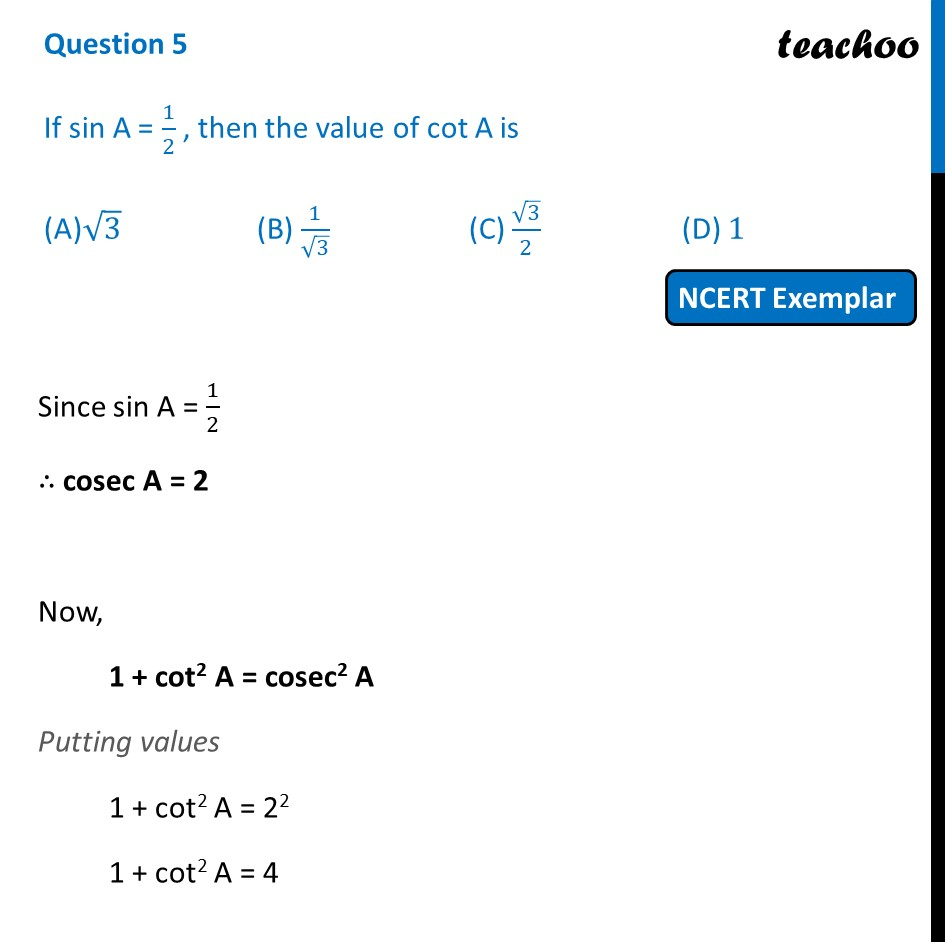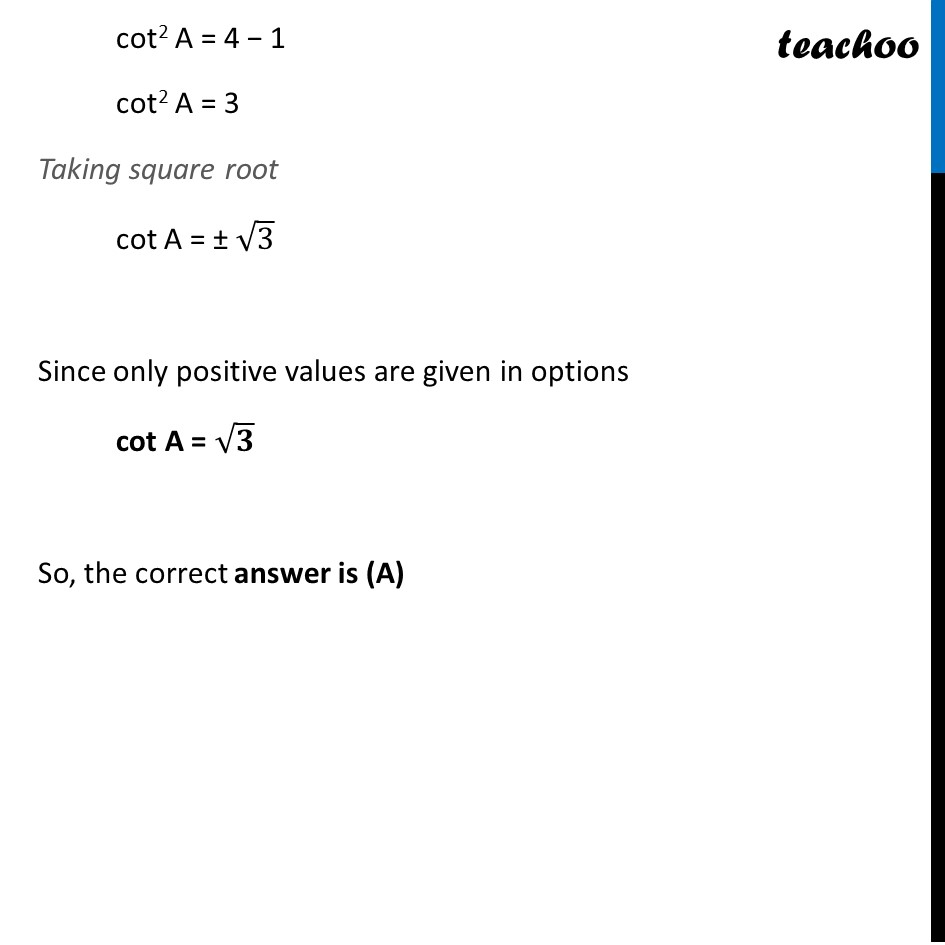NCERT Exemplar - MCQ

Chapter 8 Class 10 Introduction to Trignometry
Serial order wise

## (A)√3  (B) 1/√3  (C) √3/2  (D) 1Learn in your speed, with individual attention - Teachoo Maths 1-on-1 Class

### Transcript

Question 5 If sin A = 1/2 , then the value of cot A is (A)√3 (B) 1/√3 (C) √3/2 (D) 1 Since sin A = 1/2 ∴ cosec A = 2 Now, 1 + cot2 A = cosec2 A Putting values 1 + cot2 A = 22 1 + cot2 A = 4 cot2 A = 4 − 1 cot2 A = 3 Taking square root cot A = ± √3 Since only positive values are given in options cot A = √𝟑 So, the correct answer is (A)